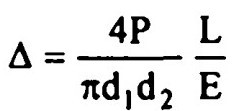Search

# Strength of Materials(361-376)

Updated: Apr 29, 2020

361. A simply supported beam with equal overhang on both sides is loaded as shown in Fig. .25

362.363.A simply supported truss shown in Fig. carries a load of 20 kN at FThe forces in the members EF and BE are respectively

zero and 10 kN (tension)

a) Both A and R are true and R is the correct explanation of A

b) Both A and R are true but R is not a correct explanation of A

c) A is true but R is false

d) A is false but R is true

364. Assertion A: In a Mohr's circle, the vertical coordinates of the ends of any

diameter are equal in magnitude and opposite in direction.

Reason R : The shear stresses on two planes at right angles are equal in

magnitude and tend to rotate the element in opposite directions a

365. Assertion A: Bending moment in a beam is maximum at a section where shear force is zero.

Reason R: Shear force at a section is given by the rate of change of bending

moment a

366. Assertion A : Strain is a fundamental behaviour of the material, while the stress is a derived concept.

Reason R : Strain does not have a unit while the stress has a unit b

367. Assertion A : The maximum bending moment occurs where the shear force is

either zero or changes sign.

Reason R: If the shear force diagram line between the two, points is horizontal,

the BM diagram line is inclined. But if the SF diagram is inclined, the.BM diagram is a parabola of second degree. b

368. Assertion A : I-section is preferred to rectangular section for resisting bending

moment.

Reason R: In I-section more than 80% of bending moment is resisted by flanges

only. a

369. Assertion A : A beam of circular cross-section in comparison to rectangular section of the same material but of equal cross sectional area can resist a larger shear force.

Reason R : The maximum intensities of shear stress in the sections of a beam of circular cross section and of a rectangular cross section are 1.33 times and 1.5 times the average shear stress respectively. a

370. Assertion A : The buckling load for a column of specified material, cross section and end conditions calculated as per Euler's formula varies inversely with the column length.

Reason R : Euler's formula takes into account the end conditions in determining

the effective length of column d

371. Assertion A : A bar tapers from a diameter of d to a diameter of d over its

length l and is subjected to a tensile force 'P' If extension is calculated based

on treating it as a bar of average diameter, the calculated extension will be more than the actual extension.

Reason R : The actual extension in such bars is given byd

372. Assertion A : Bending moment may be defined as the algebraic sum of the

moments of all forces on either side of the section.

Reason R: The rate of change of bending moment is equal to shear force at the

section. b

373. Assertion A : Macaulay's method to determine the slope and deflection at a

point in a beam is suitable for beams subjected to concentrated loads and can be

Reason R: Macaulay's method is based upon the modification of moment area

method. This is applicable to a simple beam carrying a single concentrated load

c

374. Assertion A: Rankine's theory is generally used for finding out the buckling

Reason R: Euler's theory gives higher values for buckling loads in intermediate

columns. a

375. Assertion A: A three hinged parabolic arch will not have bending moment due to uniformly distributed load.

Reason R: The vertical intercept between the linear arch and the centre line of the

actual arch gives the bending moment at a section. Due to uniformly distributed load, the linear arch will be a parabola. It will pass through the hinge and the crown. The centre line of the actual arch is also parabolic, passing through the central hinge. These two parabolas pass through three common points and hence they overlap each other a

376.Assertion A: The bending moment on a section is maximum where the shearing force changes sign.

Reason R: In a continuous curve of bending moment, the point of zero bending moment where it changes sign, is called the point of contra-flexure. b